×

Search anything:

# Time and Space Complexity of Red Black Tree

#### Time Complexity Algorithms tree data structure Data StructuresGet this book -> Problems on Array: For Interviews and Competitive Programming

In this article, we will look at the Time and Space Complexity analysis of various Red-Black Tree operations including searching, inserting, and deleting for worst, best, and average cases.

1. What is a Red-Black Tree?
2. Operations
3. Rebalancing
4. Rotations
5. Height of a Red Black Tree
6. Insertion Operation
7. Insertion Time and Space Complexity
8. Deletion Operation
9. Deletion Time and Space Complexity
10. Space Complexity
11. Conclusion

Pre-requisites:

## What is a Red-Black Tree?

A red-black tree is a balanced binary search tree whose each node is either red or black in color. Red-black trees ensure that no simple path from the root to a leaf is more than twice as long as any other by restricting the node colors, ensuring that the tree is roughly balanced.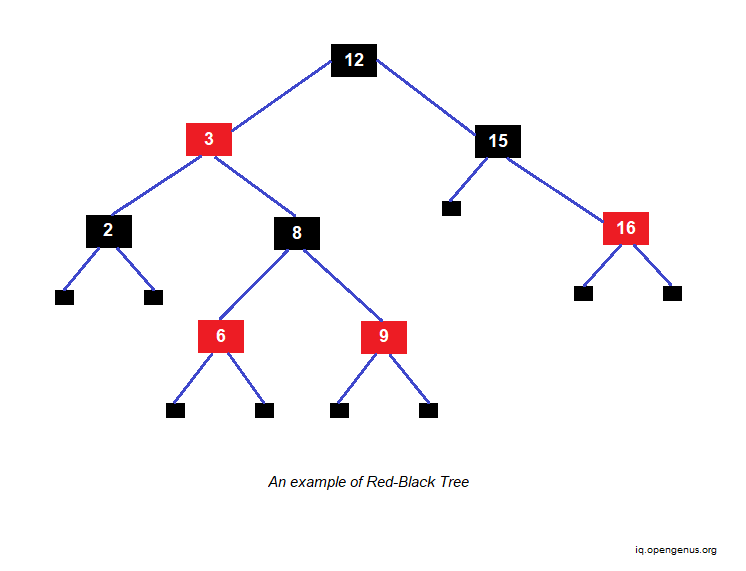Worst-case constraints for insertion time, deletion time, and search time are provided by red-black trees. This makes them valuable not only in time-sensitive applications like real-time applications, but also as basic components in other data structures that provide worst-case guarantees.

## Operations

The following is an overview of the time and space complexity of Red-Black Tree operations:

OPERATION AVERAGE CASE WORST CASE
Space O(n) O(n)
Search O(log n) O(log n)
Insert O(log n) O(log n)
Delete O(log n) O(log n)

Because every red-black tree is a particular instance of a basic binary search tree, read-only operations on a red-black tree are identical to those used for binary search trees. The immediate impact of an insertion or removal, on the other hand, may violate the red-black tree's characteristics.

## Rebalancing

The restoration of red-black tree's properties so that it becomes self-balancing is called Rebalancing. In the worst-case scenario, it requires a small amount O(log n), where n is the number of items in the tree, on average O(1), a constant number of color changes (which are quite rapid in operation), and no more than three tree rotations (two for insertion).

Despite the complexity of insert and remove operations, their timings remain O(log n).

# Rotations

In a search tree, a rotation is a local operation that preserves in-order traversal key ordering. In both trees, an in-order traversal yields the following:
A x B y C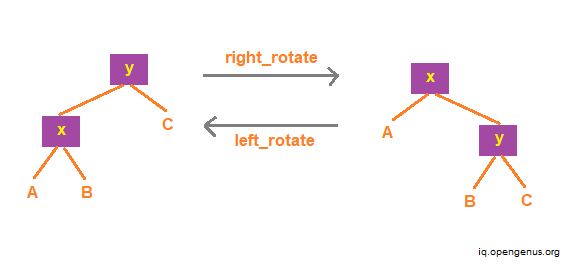The operation left rotate can be represented as follows:

``````left_rotate(Tree T, node x) {
node y;
y = x->right;
/* Turn y's left sub-tree into x's right sub-tree */
x->right = y->left;
if ( y->left != NULL )
y->left->parent = x;
/* y's new parent was x's parent */
y->parent = x->parent;
/* Set the parent to point to y instead of x */
/* First see whether we're at the root */
if ( x->parent == NULL ) T->root = y;
else
if ( x == (x->parent)->left )
/* x was on the left of its parent */
x->parent->left = y;
else
/* x must have been on the right */
x->parent->right = y;
/* Finally, put x on y's left */
y->left = x;
x->parent = y;
}
``````

These rotations can be performed in O(1) time in the best case.

# Height of a Red Black Tree

The tree's black depth is O(log nb), where nb is the number of black nodes. In order to view this:

• Imagine removing all the red nodes; the black depth would remain same, but the black depth of the leaves would now be the same as the height.
• As a result, we get a tree with the same depth of all leaves, or a full tree.
• Complete trees, as we know, have a height of O(log n).
• Since the original tree's black depth is equal to the height of the modified tree, and the height of the modified tree is O(log nb), the black depth of the original tree is O(log nb).

In the worst-case scenario, which is the situation with the tallest tree, a long path from the root to a leaf must exist. Because the number of black nodes on that lengthy path is restricted to O(log nb), having a lot of red nodes is the only option to make it longer. Because red nodes cannot have red children, the number of nodes on that path must alternate between red and black in the worst-case scenario. As a result, the path can only be twice as long as the tree's black depth. Therefore, the worst case height of the tree is O(2 log nb).
Even if the tree is completely red, nb is O(n) since only around half of the tree will be red. Therefore, the height of a red-black tree is O(log n).

As a direct result of this derivation, we can implement the dynamic set operations search, minimum, maximum, successor, and predecessor on red-black trees in O(log n) time, because each can run in O(h) time on a binary search tree of height h and any red-black tree on n nodes is a binary search tree of height O(log n).

# Insertion Operation

Insertion starts by adding the node and coloring it red, just as binary search tree insertion. In the binary search tree, we always add a leaf; in the red-black tree, leaves provide no information, therefore we instead add a red internal node with two black leaves in place of an existing black leaf. What occurs next is determined by the color of the nodes in the neighborhood. Let's see an example where we insert node z into the tree T.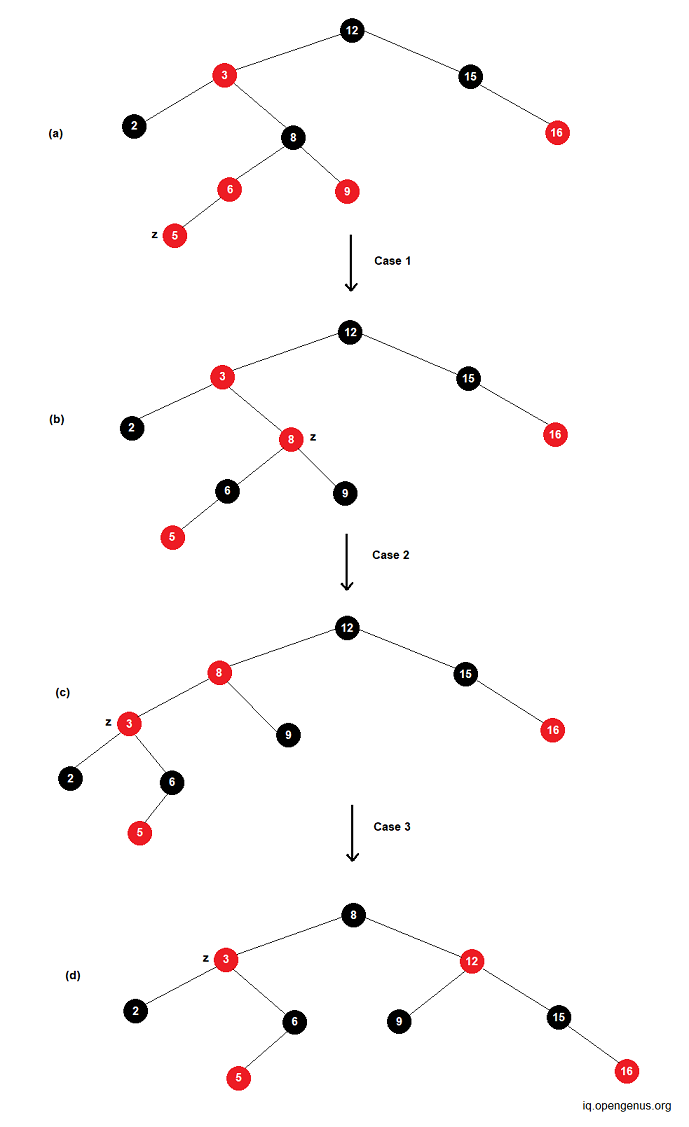## Insertion Time and Space Complexity

There are three phases to inserting a key into a non-empty tree. The binary search tree insert operation is conducted in the first phase. Because a red-black tree is balanced, the BST insert operation is O(height of tree), which is O(log n). The new node is then colored red in the second stage. This step is O(1) since it only involves changing the value of one node's color field. In the third stage, we restore any red-black characteristics that have been violated.

Changing the colors of nodes takes O(1) time. However, we may need to deal with a double-red issue farther along the route from the inserted node to the root. In the worst-case scenario, we wind up fixing a double-red condition all the way from the inserted node to the root. In the worst-case scenario, the recoloring performed during insertion is O(log n) i.e., time for one recoloring x maximum number of recoloring performed.

As a result, restoring red-black characteristics takes O(log n), and the overall time for insert is O(log n).

Best Case: In the best case, there is no rotation. Only recoloring takes place. The time complexity is O(log n). Consider the following example.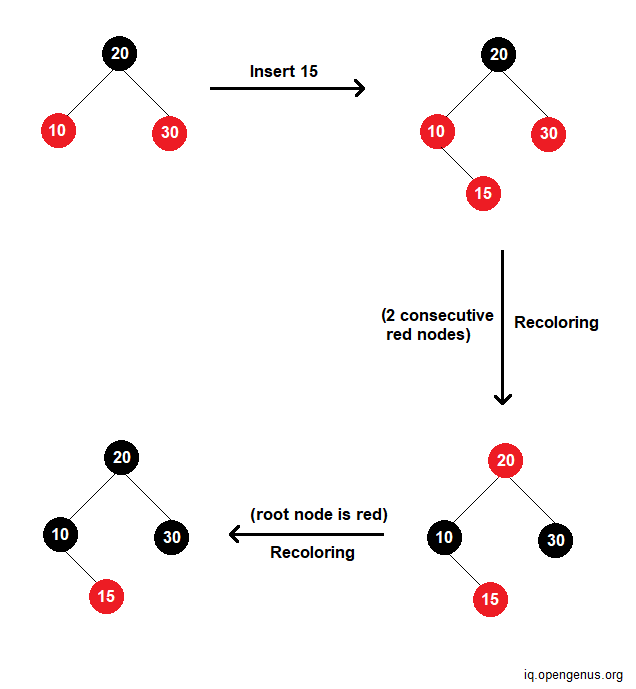Worst case: RB trees require a constant (at most 2 for insert) number of rotations. So in the worst case, there will be 2 rotations while insertion. The time complexity is O(log n). Let's see an example.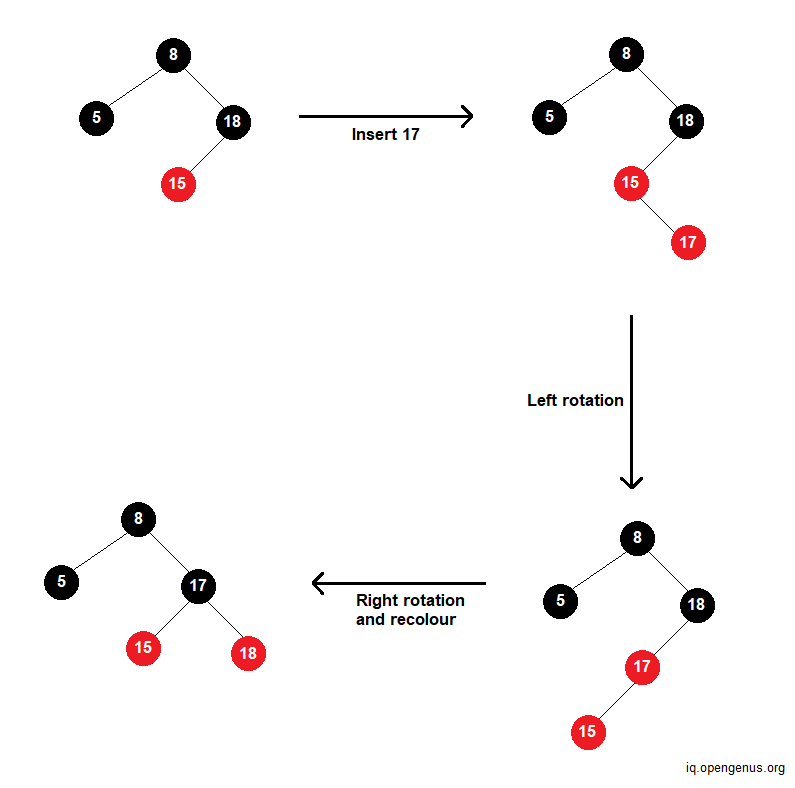Average Case: Since the average case is the mean of all possible cases, the time complexity of insertion in this case too is O(log n).

# Deletion Operation

The deletion operation in red-black tree is a little trickier than other binary trees. One thing to remember is that a red-black tree should continue be a red-black tree if an element is removed.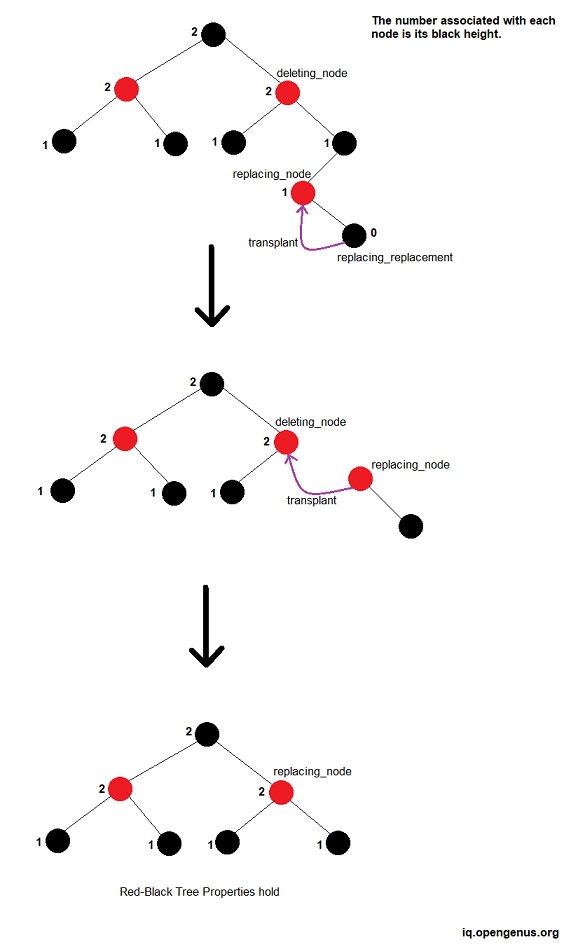## Deletion Time and Space Complexity

Finding the delete node plus the left-most right successor is proportional to the tree's height, hence it is O(log n). The swapping and deletion are both O(1). Each particular fix (rotation, for example) is O(1). In the worst-case scenario, a double-black might be transmitted up to the root. Because each rotation takes the same amount of time, this is proportional to the tree's height and so O(log n). As a result, the worst-case complexity of deletion is O(log n).

Best Case: In the best case, there is no rotation. Only recoloring takes place. The time complexity is O(log n).
Example: Delete 15 from RB tree.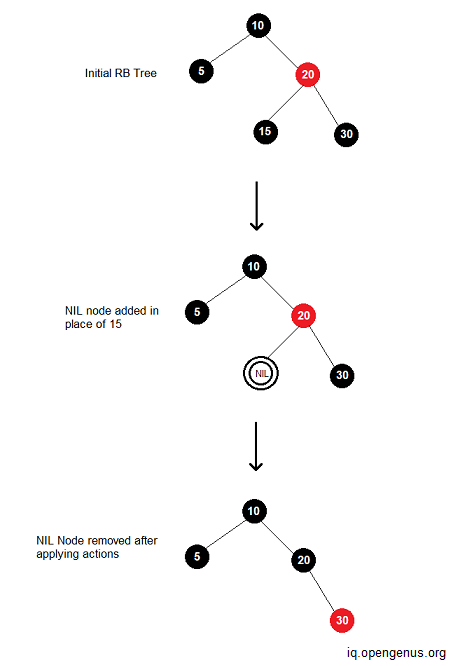Worst case: RB trees require a constant (at most 3 for deletion) number of rotations. So in the worst case, there will be 3 rotations while deletion. The time complexity is O(log n).

Average Case: Since the average case is the mean of all possible cases, the time complexity of deletion in this case too is O(log n).
Example: Delete ‘1’ from RB tree.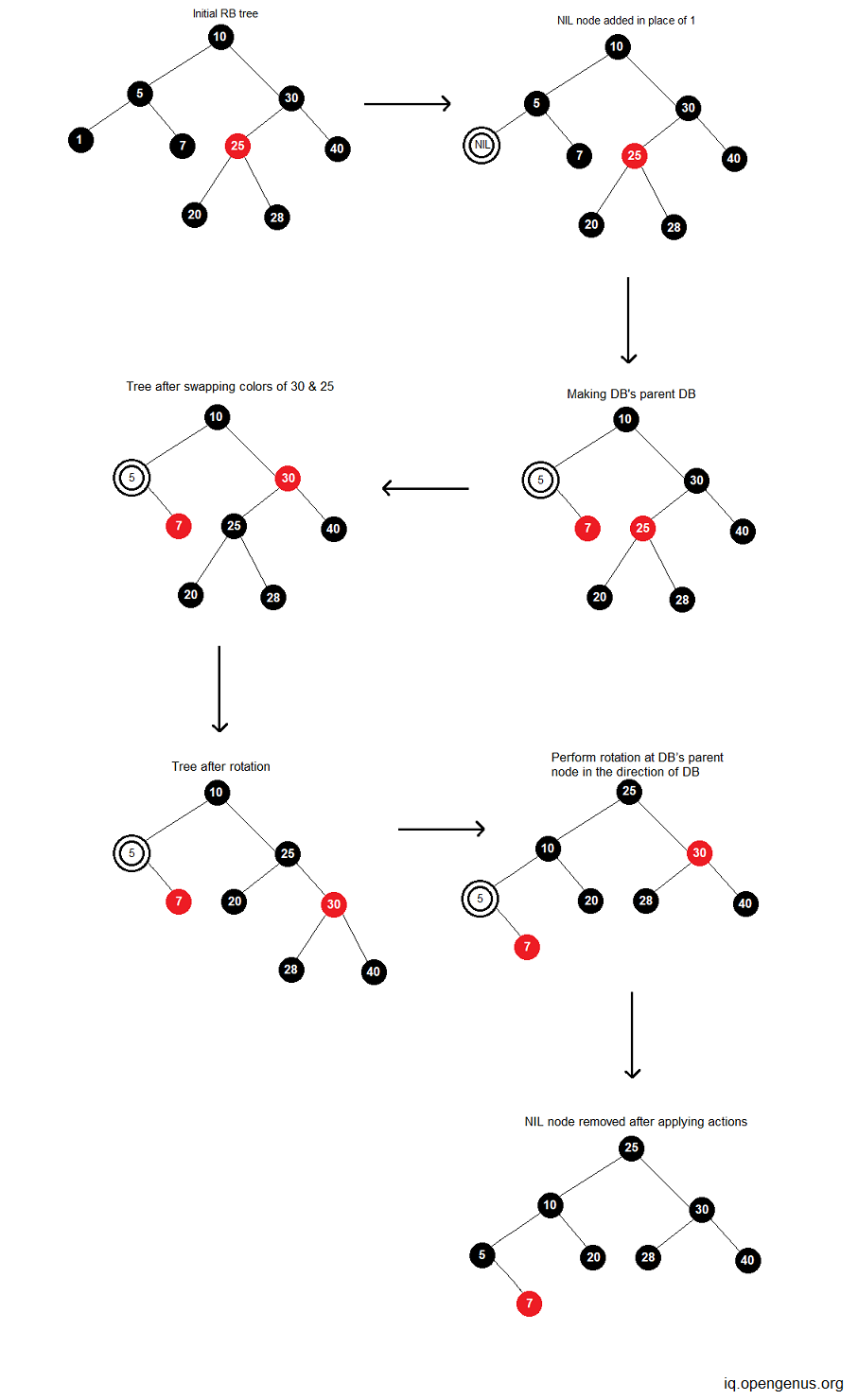# Space Complexity

The average and worst space complexity of a red-black tree is the same as that of a Binary Search Tree and is determined by the total number of nodes: O(n) because we don't need any extra space to hold duplicate data structures. We arrive to this conclusion because each node has three pointers: left child, right child, and parent. Each node takes up O(1) space. As a result, if the tree has n total nodes, the space complexity is n times O(1), which is O(n).

Because there are just two colors, monitoring the color of each node takes only one bit of information per node. Because the tree contains no extra data that distinguishes it as a red-black tree, its memory footprint is nearly comparable to that of a conventional binary search tree. In many circumstances, the extra bit of data may be stored with no extra memory cost.

# Conclusion

To conclude, all of the time and space complexities are provided below in a tabular format.

OPERATION AVERAGE CASE WORST CASE
Space O(n) O(n)
Search O(log n) O(log n)
Insert O(log n) O(log n)
Delete O(log n) O(log n)

The height of balanced search trees is always O(log n). As a result, search, insert, and delete operations on a balanced search tree may be performed in O(log n) worst-case time. Binary search trees, on the other hand, have a worst-case height of O(n), while search, insert, and delete are all O(n) in the worst-case. A balanced search tree can take several forms, including red-black trees.

Red-black trees are binary search trees that store one extra piece of information (the node's color) in each node and meet three characteristics. These attributes govern how nodes can be colored as well as the amount of black nodes along pathways leading from the root node to a null child pointer. Although it may not be visible, the procedures for restoring these attributes once a node is added or deleted result in a balanced tree.

While the search procedure for binary search trees and red-black trees is the same, the insert and delete methods for red-black trees are more sophisticated.#### Harshita Singh

Harshita Singh is pursuing her B. Tech in CSE from Harcourt Butler Technical University, Kanpur (2023). She is an Intern at OpenGenus.

Time and Space Complexity of Red Black Tree